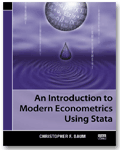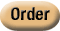»  Home »  Resources & support »  Stata news »  An Introduction to Modern Econometrics Using Stata

An Introduction to Modern Econometrics Using StataAn Introduction to Modern Econometrics Using Stata, by Christopher F. Baum, successfully bridges the gap between learning econometrics and learning how to use Stata. The book presents a contemporary approach to econometrics, emphasizing the role of method-of-moments estimators, hypothesis testing, and specification analysis while providing practical examples showing how the theory is applied to real datasets using Stata. The first three chapters are dedicated to the basic skills one needs to effectively use Stata: loading data into Stata; using commands like generate and replace, egen, and sort to manipulate variables; taking advantage of loops to automate tasks; and creating new datasets by using merge and append. Baum succinctly yet thoroughly covers the elements of Stata that a user must learn to become proficient, providing many examples along the way. Chapter 4 begins the core econometric material of the book and covers the multiple linear regression model, including efficiency of the ordinary least-squares estimator, interpreting the output from regress, and point and interval prediction. The chapter covers both linear and nonlinear Wald tests, as well as constrained least-squares estimation, Lagrange multiplier tests, and hypothesis testing of nonnested models. Chapters 5 and 6 focus on consequences of failures of the linear regression model's assumptions. Chapter 5 addresses topics like omitted-variable bias, misspecification of functional form, and outlier detection. Chapter 6 is dedicated to non–independently and identically distributed errors and introduces the Newey–West and Huber/White covariance matrices, as well as feasible generalized least-squares estimation in the presence of heteroskedasticity or serial correlation. Chapter 7 is dedicated to using indicator variables and interaction effects. Instrumental-variables estimation has been an active area of research in econometrics, and chapter 8 commendably addresses issues like weak instruments, underidentification, and generalized method-of-moments estimation. Baum uses his wildly popular ivreg2 command extensively in this chapter. The last two chapters briefly introduce panel-data analysis and discrete and limited-dependent variables. Two appendices cover importing data into Stata and Stata programming in more detail. As in all chapters, Baum presents many Stata examples. An Introduction to Modern Econometrics Using Stata can serve as a supplementary text in both undergraduate and graduate-level econometrics courses and will help students quickly become proficient in Stata. The book is also useful to economists and businesspeople wanting to learn Stata by using examples that are relevant to them. For further details or to order online, please visit the Stata Bookstore.